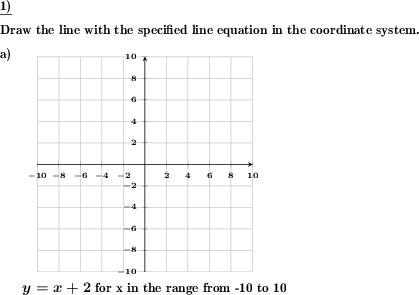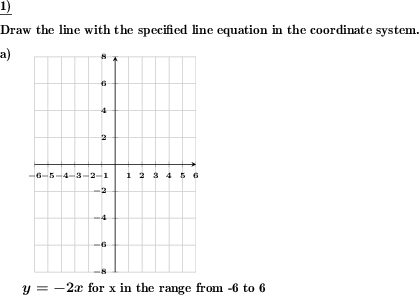Custom math worksheets at your fingertips# Details for problem "Draw a line for a given line equation"

Quickname: 9003

Elementary School, Primary School, Junior High School, Middle School, High School.

## Summary

A line equation is given, draw the respective line in a coordinate plane.

## Examples## Description

A straight line is to be drawn for a given line equation. If desired, the coordinate system can be specified or a suitable coordinate system will have to be drawn.

The straight line equation and the dimensions of the coordinate system are designed in such a way that the straight line can be drawn without particular difficulties. The Y-axis segment, for example, can be selected to lie on only full or even half coordinate plane squares (vertical divisions). It is preselectable whether the straight line may, may not or even must pass through the origin.

With regard to the coordinate system, it can be set whether only quadrant one is to be swept with positive X and Y coordinates, or also the quadrants containing coordinates with negative signs. The coordinate system can be output in three different sizes.

The slope is always such that a slope triangle would be a maximum of four boxes wide, i.e. would have a divisor of maximum four. It can be limited to only positive or only negative slope, with or without the possibility of a slope of zero.

In the straight line equation, fractions will regularly occur for m (slope) and b (Y-axis intersection). It is adjustable whether these are represented as fraction or decimal fraction.

Download free printable worksheets for this math problem here. The worksheet contains the problems only, the solution sheet includes the answers. Just click on the respective link.

•Worksheet 1Solution sheet with answers
•Worksheet 2Solution sheet with answers
•Worksheet 3Solution sheet with answers

If you can not see the solution sheets for download, they may be filtered out by an ad blocker that you may have installed. If this is the case, please allow ads for this page and reload the page. The solution sheets will then reappear.

• Do these sample worksheets do not really fit?
• Do you need more math worksheets, with a different level of difficulty?
• Would you like to combine different problems on a worksheet and adjust them to your needs?
• As a teacher, you can put together your own worksheets using the automatically generated math problems provided.
With a free initial credit, you can start creating your own math worksheets in a few minutes.

You can try it for free! Register here, to create custom worksheets now!

## Customization options for this problem

Parameter
Possible values
Number of problems
1, 2
Yes, No
Through origin
may, always, never
Slope
pos&neg or null, pos&neg not null, positive, negative
Sub-square rises
Yes, No
Size
small, medium, large
Provide square coordinate raster
Yes, No
Provide run and rise as...
fraction, decimal

## Similar problems

Remark
Description
The other way round, with the line given
For a given straight line on a coordinate plane, the corresponding equation must be specified.